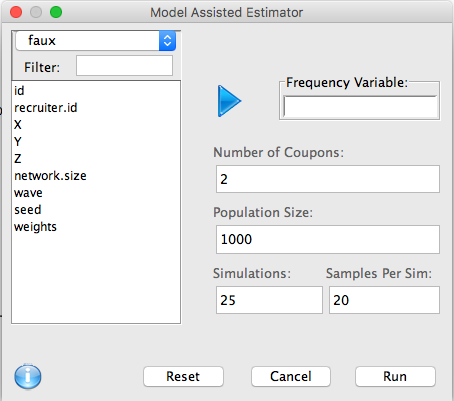The Model Assisted estimator utilizes advanced ERGM network modeling to generate an RDS corrected estimate.• ''Frequency Variable:' A categorical variable on which to perform the analysis.
• Number of coupons: The maximum number of coupons given out.
• Population size: The size of the population. Note that this procedure should only be performed on smaller populations.
• Simulations: The number of networked populations generated at each iteration.
• Samples per Sim: The number of (full) RDS samples generated for each networked population at each iteration.

The computational complexity of generating the model assisted frequency estimate grows quickly with population size, and thus this should be used only with small populations.

See Network model-assisted inference from respondent-driven sampling data for algorithm details.